### Phenomena of the Lorentz Transformation

We have learned that the Lorentz transformation of a space-time coordinate is simplest and most reasonable if the space coordinate and the time coordinate are in the same units. This is not true in our SI system. The unit of distance is one meter and the unit of time is one second.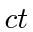for one second is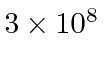meters. This is one of the reasons it was hard for us to understand transformations in the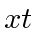plane. We would be better off with the foot and the nanosecond, but lets not do that. (Consider how messy rotations would be is we measuredin miles and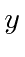in microns.)

The laws of Physics would be most easily understood if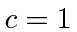but we will not make that simplification either. So we will just use the coordinatefor time. We will also have to deal with this problem for many other quantities, like Energy for example.

Working problems without a universal time is a complication to which we are not accustomed.

Consider a muon (an unstable elementary particle) that is produced by cosmic rays (mainly protons) in the upper atmosphere, with a velocity of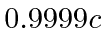. The muon has a mean lifetime of about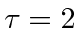microseconds (2000 feet in the units we don't use). So ignoring the Lorentz transformation, the muon could typically travel a distance ofmeters, however, with the Lorentz transformation, we will find that it can travel much farther if its velocity is near the speed of light.

Start with a frame with the muon is at rest at the origin (just as it is produced) at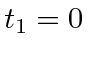. In this frame, its rest frame, it decays at, at the origin. Now we can compute the lifetime in the frame of the earth, in which the muon is moving very fast. We can also compute how far it travels before it decays. In the muon's rest frame, the earth is coming toward it with a velocity of 0.9999, meaningfor the transformation.transform to earth frame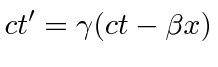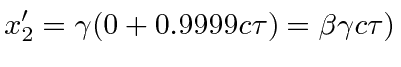decay position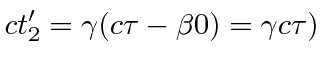decay time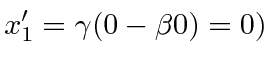prodution position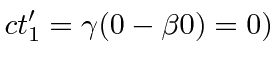production time

The lifetime viewed in the frame of the earth is, about 140 microseconds. This phenomenon is called time dilation. The distance traveled in the earth's frame iskilometers. Many of the high energy muons produced by cosmic rays can be detected as the reach the earth. They are the main component of cosmic radiation we see on the earth.

Consider a stick of lengthwith one end at the originand the other at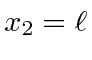meters in the unprimed coordinate system. It is at rest so thepositions of both ends is time independent in this frame. What is its length in a coordinate system moving in thedirection? How do we properly measure its length in a moving coordinate system? This problem is a little harder to solve. A reasonable definition of the length iswith the measurements made at same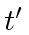, even though there is no simple way to make the measurement of both ends at the same time. The way to calculate the length is a little conterintuitive because of the restriction that we have to use the sameat each end. Lets do it for,and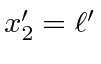. Transform back to the frame in which the stick is at rest and has a length.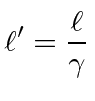Since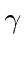is always greater than or equal to one, the stick is shorter in the moving frame. This is the phenomenon of length contraction (that Fitzgerald proposed to understand Michelson-Morley).

Let us also consider the transverse length. Since we were transforming in theplane, we assumed so far thatandare unchanged by the boost. Consider a stick of lengthalong theaxis in the rest frame. At any time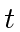, its two ends are at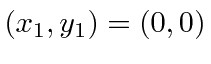and. We must face the possibility that in the primed frame, the stick will not be parallel to theaxis. At, one end will still be at the origin since bothand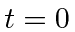. The other end will in general be at. As before, we do the transformation back into the unprimed frame. The other end of the stick will be at.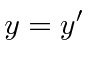Sincewe have shown thatand the stick remains parallel to the y axis. The value ofdoesn't matter because the stick is at rest in the unprimed frame. Therefore we have shown that the transverse length of the stick need not change, justifying our assumption that the transformation is in theplane and thatanddo not change under a boost in thedirection.

It is clear from these examples that Special Relativity changes our understanding of many things, including length and time. While the length of vectors is invariant under rotations, it changes under boosts. Time intervals also change when the reference frame is boosted clearly showing that there will be no way to recover an absolute time. Even Euclidean geometry is affected.

If 3 dimensional dot products and time intervals are not invariant under Lorentz boosts, what is? Lets take another look at our muon in the two frames and see if we can find anything that is invariant. In the rest frame, we have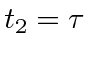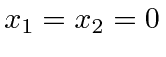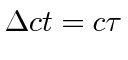In the moving frame (of the earth), we havetransformation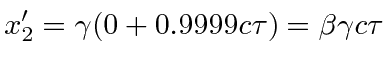decayproduction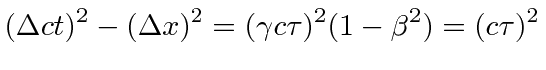This is the same as in the rest frame. At least in this case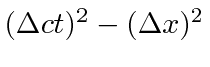is invariant under Lorentz boosts 4. It is also invariant under rotations at fixed time since it then is directly related to the 3D dot product.

Jim Branson 2012-10-21